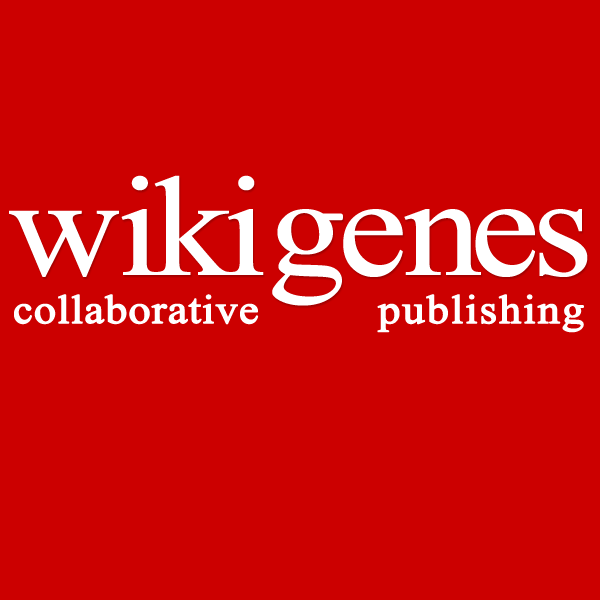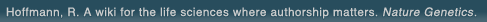# The world's first wiki where authorship really matters (Nature Genetics, 2008). Due credit and reputation for authors. Imagine a global collaborative knowledge base for original thoughts. Search thousands of articles and collaborate with scientists around the globe.

wikigene or wiki gene protein drug chemical gene disease author authorship tracking collaborative publishing evolutionary knowledge reputation system wiki2.0 global collaboration genes proteins drugs chemicals diseases compound
Hoffmann, R. A wiki for the life sciences where authorship matters. Nature Genetics (2008)# Recovery of semicircular canal primary afferent activity in the pigeon after streptomycin ototoxicity.

Recovery of semicircular canal primary afferent activity in the pigeon after streptomycin ototoxicity. J. Neurophysiol. 80: 3297-3311, 1998. The electrophysiological activity of horizontal semicircular canal primary afferents (HSCPA) was investigated in vivo in the barbiturate-anesthetized pigeon by means of extracellular single-fiber vestibular nerve action potential recordings. The spontaneous and driven discharges to pulse (step/trapezoid waveform, peak velocity = 120 degrees/s) and sum-of-sines (0.03, 0.09, 0.21, 0.39, 0.93, 1.83 Hz, peak velocity = 30 degrees/s for each frequency) rotations were measured both in normal control animals and a group of animals at 30, 40, 50, 60, 71, and 150 days postinjection sequence (PIS) of streptomycin sulfate. Prior to 30 days PIS, the activity in the nerve was not appropriately modulated during and after rotation. At 30 days PIS and thereafter, the responses resembled those observed in control animals but with systematic changes in parameters of fitted pulse responses and fitted Bode plots as days PIS increased. The return of parameters characterizing the neural dynamics of the semicircular canals were monotonic and could be best described by either linear or exponential functions. After 30 days PIS, the mechanical cupula-endolymph system, the function of which can be inferred from the cupula long time constant (tauL) following step velocity, did not change systematically (tauL = 6.92 +/- 3.96, 8.64 +/- 5.52, 8.35 +/- 4.21, 10.00 +/- 2.79, 9.05 +/- 3.67, 7.05 +/- 2.72; means +/- SD). However, the mean gain (G) of the HSCPA response to pulse rotation nearly doubled between 30 and 150 days PIS (from 1.31 +/- 0. 39 to 2.40 +/- 1.04) and returned linearly to control values (G = 2. 39 +/- 0.77) over this time period [G = 1.33 + 0.009(PIS-30), R2 = 0. 92, P < 0.05]. Meanwhile, neural adaptation as quantitated using a fractional operator, k, decayed exponentially (single exponential) to an asymptote. The time constant of this exponential was approximately 55 days [k = 0.034 + 0.33e-(PIS-30)/55.4, R2 = 0.99, P < 0.01]. Features of the spontaneous discharge previously shown to be correlated with k changed appropriately. That is, the coefficient of variation (CV) and frequency of firing (FF) decayed and grew asymptotically, respectively. These parameters also exhibited an exponential time course of return to control values from 30 to 150 days PIS [CV = 0.44 + 0.65e-(PIS-30)/21.5, R2 = 0.96, P < 0.01, and FF = 39.97 + 101.42(1 - e-(PIS-30)/32.6), R2 = 0.97, P < 0.01]. The trends of recovery for G, k, and tauL derived from analysis of the pulse response were confirmed by strong positive correlations with best fitted parameters obtained from analysis of the sum-of-sines frequency domain response of HSCPAs. There were statistically significant correlations (r = 0.90, P < 0.05 and r = 0.93, P < 0.05) between parameters (G, k) derived from pulse responses and those (G', k') from sum-of-sines responses, respectively. The cupula time constant based on sum-of-sines' data (tau'L) showed no statistically significant change between 30 and 150 days PIS (P > 0.05, analysis of variance). Thus the results in present study indicate that both the spontaneous discharge and the driven response to rotation of pigeon HSCPAs recovered their normal physiological status between 30 and 150 days PIS after hair cell death due to aminoglycoside ototoxicity. The recovery was systematic for the parameters chosen to be tested with the exception of the cupula long time constant, tauL. The mechanisms (changes in ciliary dynamics, changes in hair cell ionic currents, changes in bouton terminals, etc.) underlying these changes await further morphophysiological studies.